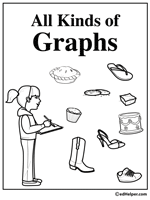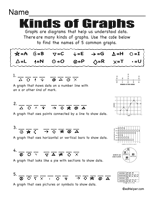Worksheets and No Prep Teaching Resources
Math Worksheets

# Analyze Data and Graphs Worksheets

Starting young mathematicians early on the road to understanding and analyzing graphs is a smart move because they will advance miles when they reach higher levels of math. These innovative worksheets for grades one through four and up will help students understand pictographs, vertical and horizontal bar graphs, and circle graphs with ease. Since graphs show information in a friendly, understandable way, these worksheets can help students develop excellent visual and communication skills for life.All Kinds of Graphs

Graphs Activity BookGraph Puzzles

Surveys

Graphs Tool - Create your own graphs and printables
Create your own graphs and printables

Data, Graphs, and Picture Graphs (grades 2-3)
Picture Graphs
Picture graphs and questions
Use the data to make a horizontal bar graph
Use the data to make a vertical bar graph
Table Graphs (with pictures)
Table graphs and questions
Use the data to make a horizontal bar graph
Use the data to make a vertical bar graph
Tally Charts
Tally charts and questions
Use the data to make a horizontal bar graph
Use the data to make a vertical bar graph
Mixed Review
Data, Graphs, and Picture Graphs Mixed Review

GlyphsGraphs Glyph

Glyphs

Science Project
A Picture Is Worth a Thousand Words

Graph and Analyze Data (introduction; grades 3 and up)
Tally table
Frequency table
Make a bar graph from a tally table
Make a bar graph from a frequency table
Line plots
Stem-and-leaf plots
Vertical bar graphs
Vertical bar graphs (draw graph from table)
Horizontal bar graphs
Horizontal bar graphs (draw graph from table)
Ordered pairs: Write the ordered pair for each point
Ordered pairs: Find each ordered pair. Write the letter for the point named by the ordered pair.
Ordered pairs: Draw the ordered points on the grid. Label each point.
Line graphs
Line graphs (draw graph from table)
Graph and Analyze Data Mixed Review

Graph and Analyze Data (more questions for grades 4 and up)
Stem-and-leaf plots
Vertical bar graphs
Horizontal bar graphs
Circle graphs
Graph and Analyze Data Mixed Review

Mean, Median, and Mode

Line Plots
Calculating range from a line plot
Calculating mode from a line plot
Calculating median from a line plot
Calculating mean from a line plot
Mixed

Stem-and-Leaf Plots
Calculating range from a stem-and-leaf plots
Calculating mode from a stem-and-leaf plots
Calculating median from a stem-and-leaf plots
Calculating mean from a stem-and-leaf plots
Mixed

5-7 Numbers
Find the range
Find the mean
Find the median
Find the mode
Find the mean, median, and mode
Find the value
Fine the missing number

8-13 Numbers
Find the range
Find the mean
Find the median
Find the mode
Find the mean, median, and mode
Find the value
Fine the missing number

Review
Mean, Median, and Mode Mixed Review

More Activities and Printables
 Graphing

Teacher Recommendations:  Worksheet and Lesson Plan Activity Ideas

Math

Money

Columbus Day

Cause And Effect

Maps

Pumpkins

Science

Fire Safety

Spiders

Weather

Writing